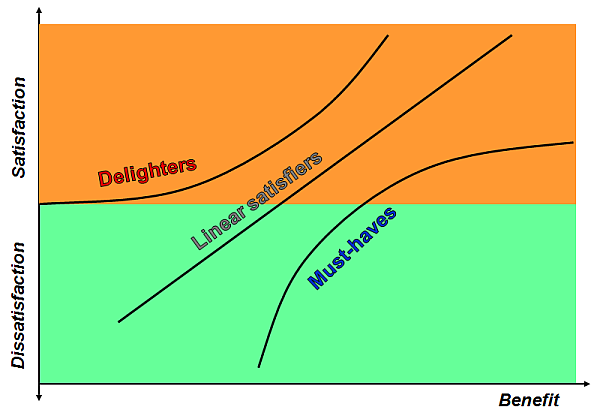## Formal classification

Four basic classification scales of measurement variables are commonly used in market research [Stevens, 1951].

• Nominal scaled
Every value is a code for a numerically incomparable entity, such as outlet, make, brand or color. The values have no natural order and allow no mathematical operations.
• Ordinal scaled
A value gives a ranking of the item, e.g. version or grade of a product, top-speed, loading capacity, etc. There is no distance defined between the scale points.
Some values may be skipped and not used as levels, e.g. when a too "dense" scale needs to be simplified.
An interval-based variant of the scale is often obtained from a quantitative variable, often when its distribution is unknown. An advantage is truncation of the distribution tails, e.g. for the stated income level. .
• Interval scaled
The difference between consecutive values is equal, but the base or reference point, i.e. the zero value, is arbitrary and/or set by a convention.
The values can be shifted (a constant added), counted up, multiplied by a constant (re-scaled) but not multiplied mutually. An example may be temperature that can be given in Celsius, Fahrenheit, Kelvin or Rheomir degrees.
• Ratio scaled
Values can be either integers, e.g. number of pieces, or decimal values. The most distinct property is existence of a non-arbitrary, uniquely defined zero representing the absence of the object or value.
Type of variable scaling Values can be rank-ordered Values can be separated by equal interval Non arbitrary zero
Nominal - - -
Ordinal X - -
Interval X X -
Ratio X X X

Variables with higher level of measurement scaling can always be transformed (or recoded) to a lower level of measurement scaling. This is often the case when distribution of the original quantity is strongly asymmetric or heavy tailed which excludes use of methods that assume normal or any other "well behaved" distribution of random variables. This is especially true for most of linear methods of classical statistics. E.g., family income is often recoded into ordinal categories based on census. More or less heuristic Likert scales are often used to get rid of heavy tails.

## Behavioral classification

On the real market, purchasers seldom make optimal choices. A choice of goods is often a compromise. It is believed that the most efficient way for a consumer to accomplish a purchase is a "satisficing", i.e. choosing the first item that is
• sufficient and
• fairly satisfying.
If none of the above conditions is met the purchase will not proceed. In CBC - Choice Based Conjoint, this situation is simulated by a choice of a constant alternative, mostly a "none of these" one.As aside
• Satisficing is due to incomplete knowledge or awareness, inactivity, or lack of time or expectation on the side of the purchaser, or poor accessibility of an optimal product at the time of the actual purchase. However, indirect utility of the chosen product is often close to that of an optimal product. This should be respected in what-if simulation and its interpretation.
• Satisficing is closely bound to affordability. An attractive product that has high "felicity" but is not affordable has low preference, and, therefore, low indirect utility and choice probability. It is this probability we are interested in.
• A possible analytic approach to satisficing is estimation of the calibrated product acceptance and the competitive potential derived from it, especially in combination with a behavioral segmentation of the target.

An interval or ratio scaled market variable can, and in most cases does, contribute to satisfaction with a product in a monotonic way. However, the increment is seldom directly proportional to the change of the marketing variable. Utility dependence on a quantity (or a quality given by a value) of a benefit can be of four basic types:

• Must-haves, typically with concave dependence.
• Delighters (latent needs) , typically with convex dependence.
• Linear satisfiers, i.e. with linear dependence on the [possibly transformed] value.
• Neutrals have no or undetectable influence.As aside
• The picture is a simplification of Kano diagram used in Quality Function Deployment.
• An analogous reasoning can be used for detriments (inverted benefits), e.g. for price attributes (fees, charges, rates, tariffs, etc.).
• Any monotonic transformation of a utility is a utility on a different interval scale. A monotonic transformation of a quantitative benefit attribute is often useful to obtain a "linear satisfier". This is often possible with price attributes under certain conditions.